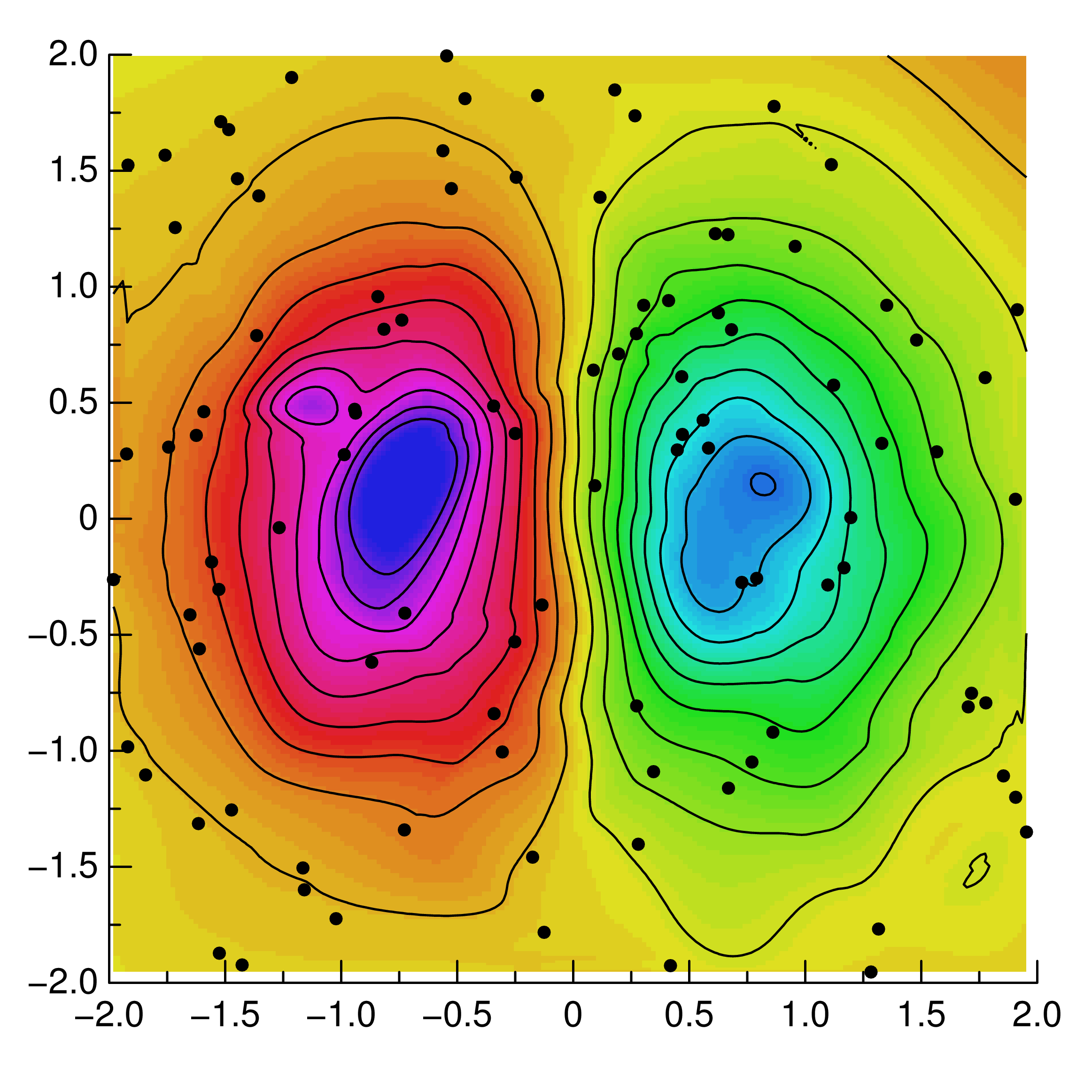# Using the GR framework in Fortran¶

As a C library GR can not only be used directly in C and C++ or with a wrapper in Python and Julia, but it can also be called from Fortran, e.g. using the GNU Fortran compiler.

When using GR in Fortran, you will need to define the GR and GKS integer constants yourself, e.g. for GKS_K_MARKERTYPE_SOLID_CIRCLE as seen below, and it is important to use double precision constants for floating point parameters. Aside from these caveats, you can refer directly to the API reference for the C library GR.

The following example shows how GR can be used in Fortran to create a contour plot of unstructured two dimensional data:

C
C   gfortran griddata.f -L/usr/local/gr/lib -lGR
C
program griddata
C
integer GKS_K_MARKERTYPE_SOLID_CIRCLE
parameter (GKS_K_MARKERTYPE_SOLID_CIRCLE = -1)
C
double precision xd(100), yd(100), zd(100)
double precision x(200), y(200), z(200, 200)
double precision h(20)
integer i
C
call srand(0)
do 1 i = 1, 100
xd(i) = -2 + 4 * rand()
yd(i) = -2 + 4 * rand()
zd(i) = xd(i) * exp(-xd(i)*xd(i) - yd(i)*yd(i))
1   continue
C
call gr_setviewport(0.1D0, 0.95D0, 0.1D0, 0.95D0)
call gr_setwindow(-2.0D0, 2.0D0, -2.0D0, 2.0D0)
call gr_setspace(-0.5D0, 0.5D0, 0, 90)
call gr_setmarkersize(1.0D0)
call gr_setmarkertype(GKS_K_MARKERTYPE_SOLID_CIRCLE)
call gr_setcharheight(0.024D0)
call gr_settextalign(2, 0)
call gr_settextfontprec(3, 0)
C
call gr_gridit(100, xd, yd, zd, 200, 200, x, y, z)
do 2 i = 1, 20
h(i) = -0.5 + i / 19.0
2   continue
C
call gr_surface(200, 200, x, y, z, 5)
call gr_contour(200, 200, 20, x, y, h, z, 0)
call gr_polymarker(100, xd, yd)
call gr_axes(0.25D0, 0.25D0, -2D0, -2D0, 2, 2, 0.01D0)
C
call gr_updatews()
end


The result will be exactly the same as if you had used a different programming language, except for slight differences due to the underlying random number generators.